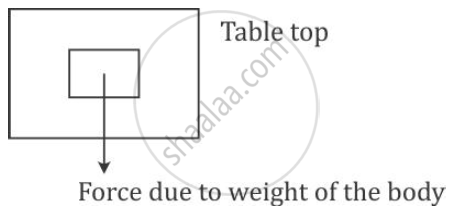Share

# When a Body is Placed on a Table Top, It Exerts a Force Equal to Its Weight Downwards on the Table Top but Does Not Move Or Fall Name the Force Exerted by the Table Top and What is the Direction of the Force? - ICSE Class 10 - Physics

ConceptWork, Energy, Power - Relation with Force

#### Question

When a body is placed on a table top, it exerts a force equal to its weight downwards on the table top but does not move or fall1) Name the force exerted by the table top

2) What is the direction of the force?

#### Solution

1) The normal reaction force is exerted by the table top.

2) The force is in the upward direction.

Is there an error in this question or solution?

#### APPEARS IN

Solution When a Body is Placed on a Table Top, It Exerts a Force Equal to Its Weight Downwards on the Table Top but Does Not Move Or Fall Name the Force Exerted by the Table Top and What is the Direction of the Force? Concept: Work, Energy, Power - Relation with Force.
S